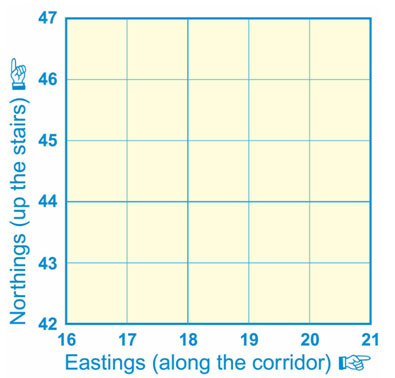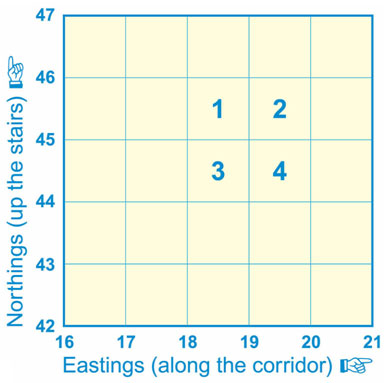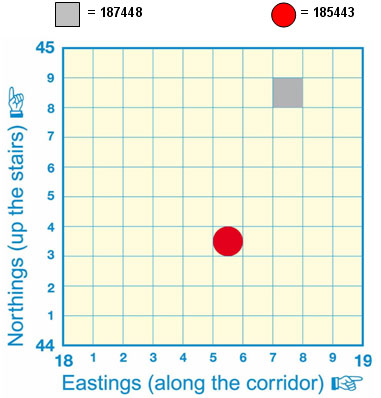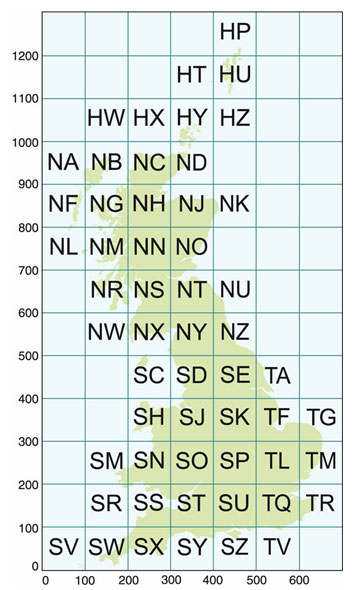# How to plot a National Grid Reference

Ordnance Survey maps are covered in a series of faint blue lines that make up a grid. The lines have numbers accompanying them that allow you to accurately pinpoint your location on a map. Once you have located where you are, the grid system makes it simple to give others (such as mountain rescue) an accurate description of your location. This description, which will be a series of numbers, is known as a grid reference.

Grid references

Before you begin to look at grid references it is important to be aware that all the numbers going across the face of the map, for example, left to right, are called eastings (this is because they are heading eastward), and similarly, all the numbers going up the face of the map from bottom to top are called northings again because they are heading in a northward direction).

There are two main types of grid reference:

• 4-figure - for example, 1945, this indicates a single kilometre square on an Ordnance Survey map.
• 6-figure - for example, 192454, shows a point within a square.Figure 11: Example of an Ordnance Survey grid system

4-figure map references

When giving a 4-figure grid reference you should always give the eastings number first and the northings number second, very much like when giving the reading of a graph in school - you must go along the corridor/hallway (horizontal) and then up the stairs (vertical).

For example, the number 2 in the diagram below is 19 across and 45 up and therefore the 4-figure grid reference is 1945.Figure 12: Theoretical points in grid square

The numbered squares on figure 12 above would have the following 4-figure grid references:

1 = 18 45        2 = 19 45

3 = 18 44        4 = 19 44

6-figure map references

Having worked out the basic 4-figure grid reference, for example, square 3 below, imagine this square is further divided up into tenths (see figure 13). Using the example below, the grey box is in the square 1844. More accurately it is 7 tenths across and 8 tenths up within the grid square 1844 and therefore has the 6-figure map reference 187448.

The shapes on the map below would have the following 6-figure grid references:Figure 13: Square 3 divided into tenths

National Grid Lines

As well as numbered grid lines, Ordnance Survey maps have codes made of two letters. These two letter codes can be found printed in faint blue capitals on Ordnance Survey maps. The whole of Great Britain is divided into squares of 100 km and each square is given two letters (see figure 14). There will be a diagram within your map's key showing you which areas of your map fall into different squares of the National Grid.

When you quote your six-digit grid reference you should put the two letters of the area you are in before the numbers. This means that there is no doubt or confusion about your location. For example, you may be at grid reference 509 582 in south-west Scotland. The complete grid reference you should quote would be NX 509 582 (without the letters the numeric reference would be repeated in every 100 km square).Figure 14: The grid layout of Great Britain

Reproduced with kind permission from Ordnance Survey (January 2007). © Crown Copyright